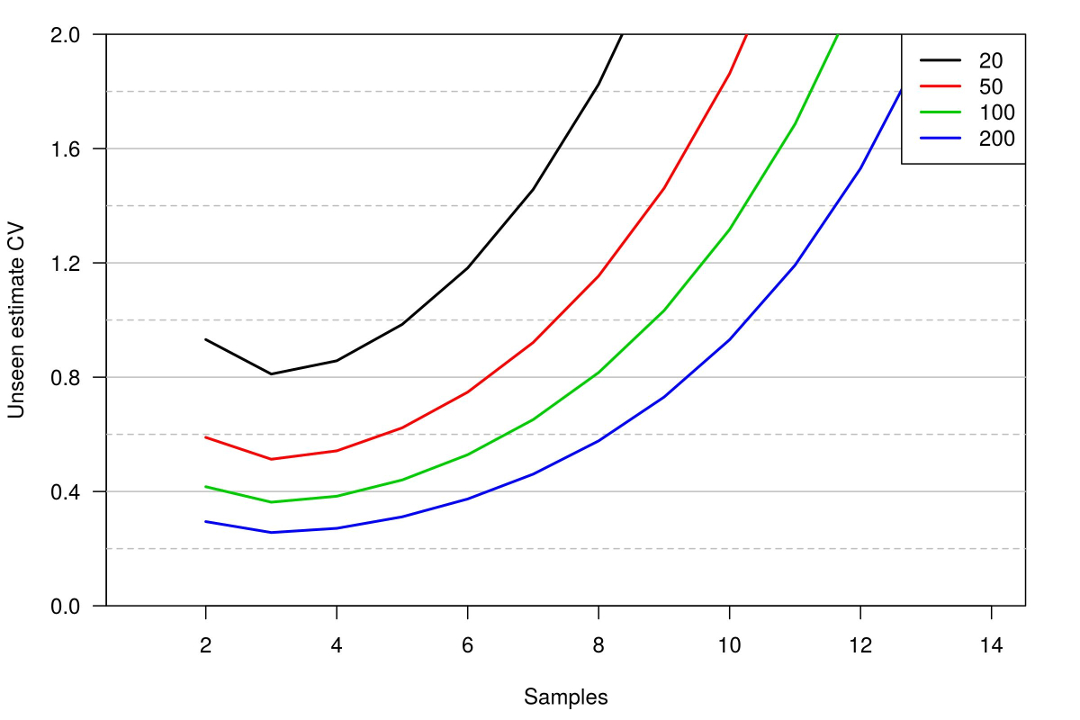In this video Darryl provides some tips on sample size considerations for estimating abundance or population size with closed population capture-recapture (or mark-recapture) methods. Here is the R code used to create the plots discussed in the video.

When capture probability is assumed to be constant (i.e., M0), the standard error for the estimate of abundance can be calculated using this function, for given values of N (abundance), p (capture probability) and t (the number of sampling occasions):

```SE_N<-function(N,p,t){
return(sqrt(N/((1-p)^-t-t/(1-p)+t-1)))
}```

This is also the standard error for the estimated number of individuals in the population that are not captured (which is the fundamental quantity we need to estimate, how many additional individuals are in the population that were not captured). The number not captured is often denoted as f0.

```N<-c(20,50,100,200)
t<-2:14
p<-0.2

# Create an array of standard error values with different values of
# N and t, when p=0.2.
seN<-outer(N,t,function(a,b) {SE_N(a,p,b)})

# Calculate the expected number of individuals captured at least once,
# and expected number never captured.
EMt_1 <- outer(N,t,function(a,b){a*(1-(1-p)^b)})
Ef0<-N-EMt_1

# Calculate the CV for N and f0
CVN<-seN/N
CVf<-seN/Ef0```

Now plot the CVs and expected number of individuals captured at least once.

```# Plot the CV of f0
plot(t,CVf[1,],ylim=c(0,2),yaxs="i",type="n",las=1,lwd=2,
xlim=c(1,14),xlab="Samples",ylab="Unseen estimate CV",
main="p = 0.2",yaxt="n")
axis(2,at=seq(0,2,0.4),las=1)
abline(h=seq(0.2,1.8,0.2),col="grey",lty=2:1)
for(ii in 1:4){
points(t,CVf[ii,],type="l",lwd=2,col=ii)
#  text(x=1.3,y=CVN[ii,1],labels=paste("p=",N[ii]))
}
legend("topright",legend=N,lwd=2,col=1:4)

# Plot the CV of N
plot(t,CVN[1,],ylim=c(0,1),yaxs="i",type="n",las=1,lwd=2,
xlim=c(1,14),xlab="Samples",ylab="Abundance estimate CV",
main="p = 0.2")
abline(h=seq(0.1,0.9,0.1),col="grey",lty=2:1)
for(ii in 1:4){
points(t,CVN[ii,],type="l",lwd=2,col=ii)
#  text(x=1.3,y=CVN[ii,1],labels=paste("p=",N[ii]))
}
legend("topright",legend=N,lwd=2,col=1:4)

# Plot the expected number captured at least once
plot(t,EMt_1[1,],ylim=c(0,200),yaxs="i",type="n",las=1,lwd=2,
xlim=c(1,14),xlab="Samples",ylab="Expected number captured",
main="p = 0.2")
abline(h=seq(10,190,10),col="grey",lty=2:1)
for(ii in 1:4){
points(t,EMt_1[ii,],type="l",lwd=2,col=ii)
#  text(x=1.3,y=CVN[ii,1],labels=paste("p=",N[ii]))
}
legend("topleft",legend=N,lwd=2,col=1:4)```